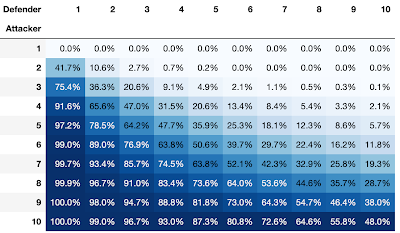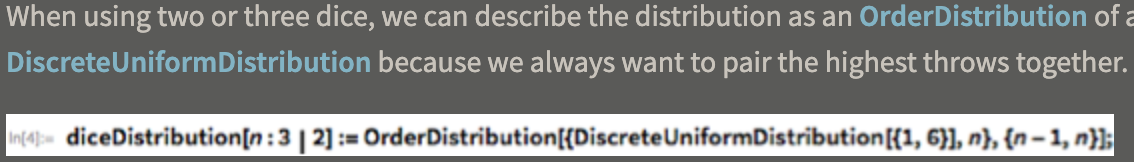## 1 Risk

### 1.1 The Game

• Domain: the dice battles, not the board game
• Attacker and Defender each have some number of units
• Units battle with die rolls in successive rounds
• Attacker goal: destroy all Defender units
• Defender goal: reduce Attacker units to 1

### 1.2 Battle Unit Rules

• Attacker has A units, Defender has D units
• Attacker can use min(3, A-1)
• Defender can use min(2, D)

### 1.3 Battle Dice Rules

• A and D rolls are sorted in descending order and zipped
• e.g. A {4,1,3} and D {4,2} -> [(4,4), (3,2)]
• Defender has advantage: win on condition (>=)
• e.g. (4,4) -> Defender wins if 4 >= 4

### 1.4 Question

• Given starting number of units (A, D)…
• What is the exact probability that Attacker wins?

## 2 Computing Probabilities

### 2.1 Approach

• Find out probability of each possible battle outcome
• Chain probability of outcomes
• From game specs, there are limited battles
• [(3,2), (3,1), (2,2), (2,1), (1,2), (1,1)]

### 2.2 Outcome Example

• (1,1): Attacker 1 die vs Defender 1 die
• What are outcomes and associated probabilities?
• Both die can roll 1 to 6, so 36 battle permutations
• Attacker loses in 21 / 36; Defender loses in 15 / 36

### 2.3 Haskell Implementation

``````successExact :: Battlefield -> Probability
successExact (Battlefield _ 0) = 1
successExact (Battlefield 1 _) = 0
successExact b =
case M.lookup (maxTroops b) pMap of
Nothing -> 0
Just ps -> agg \$ map update \$ filter (not . aLoses b) ps
where update (p, losses) = (p, updateField b losses)

agg :: [(Probability,  Battlefield)] -> Probability
agg pairs = foldr f 0 pairs
where f (p, b) accP = accP + (p * successExact b)
``````

## 3 Results

### 3.1 10 x 10 Matrix### 3.2 Notes

• The Rational type in Haskell gives exact results
``````A: 3 B: 3 | Exact: 20.61%  | Exact: 692225 % 3359232
A: 3 B: 4 | Exact: 9.13%   | Exact: 5520775 % 60466176
``````

### 3.3 Also Interesting

• Wolfram Alpha### 3.4 Next Steps

• Elm visualization of probability chain!